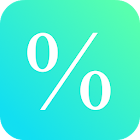Percent Calculator

• 검색 유형

모든 Android 애플리케이션 카테고리

모든 Android 게임 카테고리# Percent Calculator

by: 1.3K 8.4

8.4 사용자
평가 등급

## 스크린샷

설명

This free math calculator lets you calculate percentages from a real number.

Best mathematical tool for school and college! If you are a student, it will helps you to learn percent calculations with numbers.

Note: In mathematics, a percentage is a number or ratio expressed as a fraction of 100. It is used to calculate increase and decrease, concentration, interest and so on.

: 1.341 리뷰

"우수"

8.4